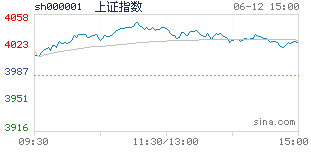# 新网站上线庆祝活动公告  已结束##### var date = ("2016-01-01 00:00:00"); var dateTime = date.split(" "); for(var i =0;i<dateTime.length;i++){ var date = dateTime+""; var ymd = date.split("-"); for(var j =0;j<ymd.length;j++){ var y = ymd+"年"; var m = y+ymd+"月"; var d = m+ ymd+"日 "; } } var hm = dateTime.substring(0,dateTime.lastIndexOf(":")); var time = d+hm; \$(".startDate1").html(time); - var date = ("2016-01-31 23:59:59"); var dateTime = date.split(" "); for(var i =0;i<dateTime.length;i++){ var date = dateTime+""; var ymd = date.split("-"); for(var j =0;j<ymd.length;j++){ var y = ymd+"年"; var m = y+ymd+"月"; var d = m+ ymd+"日 "; } } var hm = dateTime.substring(0,dateTime.lastIndexOf(":")); var time = d+hm; \$(".endDate1").text(time);### 第二届(2015)中国期货资管精英大会  已结束

--

## 每日财经速递

• 上证综指
• 3632.88
• -14.01
• -0.38%